### Discover more content...

Enter some keywords in the search box above, we will do our best to offer you relevant results.

### We're sorry!

We couldn't find any results for your search. Please try again with another keywords.

### 一. 原始值和对象

1.原始值：BOOL，Number，String，null，undefined。
2.对象：每个对象都有唯一的标识且只严格的等于( = = = ) 自己。

null，undefined没有属性，连toString( )方法也没有。

false，0，NaN，undefined，null，' ' ，都是false。

typeof 运算符能区分原始值和对象，并检测出原始值的类型。
instanceof 运算符可以检测出一个对象是否是特定构造函数的一个实例或者是否为它的一个子类。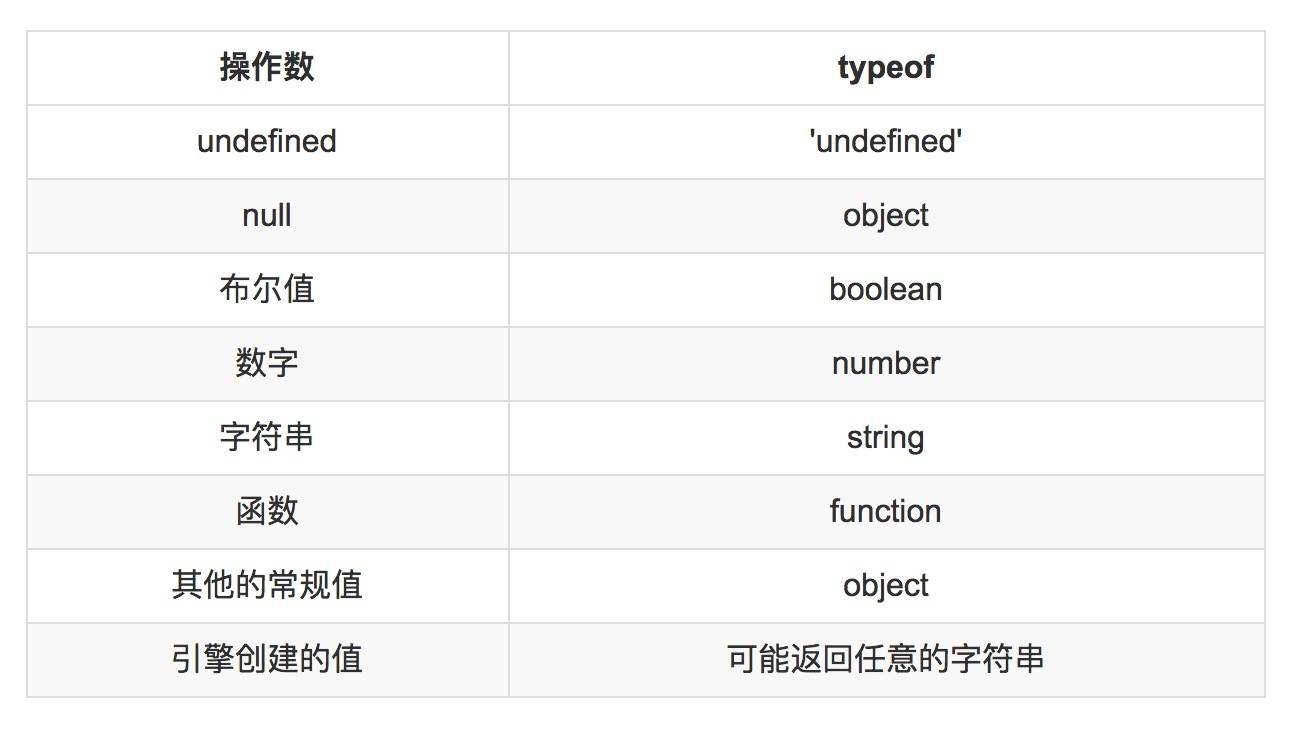null 返回的是一个 object，这个是一个不可修复的 bug，如果修改这个 bug，就会破坏现有代码体系。但是这不能表示 null 是一个对象。

``````
function isObject (value) {
return ( value !== null
&& (typeof value === 'object'
|| typeof value === 'function'));
}

``````

null 是原型链最顶端的元素

``````
Object.getPrototypeOf(Object.prototype)

< null

``````

``````
if(x === null) {
// 判断是否为 null
}

if (x === undefined) {
// 判断是否为 undefined
}

if (x === void 0 ) {
// 判断是否为 undefined，void 0 === undefined
}

if (x != null ) {
// 判断x既不是undefined，也不是null
// 这种写法等价于 if (x !== undefined && x !== null )
}

``````

``````
NaN === NaN
<false

``````

``````
// 原始值转换成对象
var object = new String('abc')

// 对象转换成原始值
String(123)
<'123'

``````

``````
new Boolean(true).valueOf()
<true

``````

``````
Boolean(new Boolean(false))
<true

``````

### 二. 宽松相等带来的bug

1. 严格相等 ( = = = ) 和严格不等 ( != = ) 要求比较的值必须是相同的类型。
2. 宽松相等 ( = = ) 和宽松不等 ( ！ = ) 会先尝试将两个不同类型的值进行转换，然后再使用严格等进行比较。

``````
undefined == null // undefined 和 null 是宽松相等的
<true

2 == true  // 不要误认为这里是true
<false

1 == true
<true

0 == false
<true

' ' == false // 空字符串等于false，但是不是所有的非空字符串都等于true
<true

'1' == true
<true

'2' == true
<false

'abc' == true // NaN === 1
<false

``````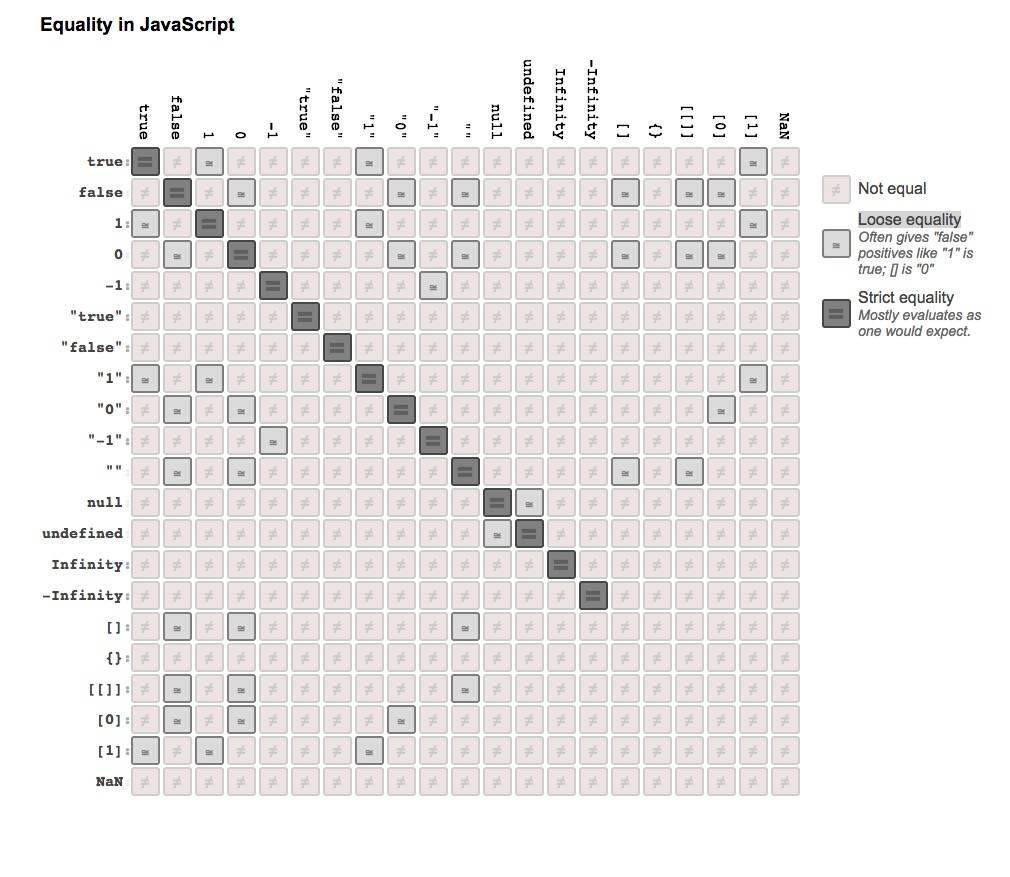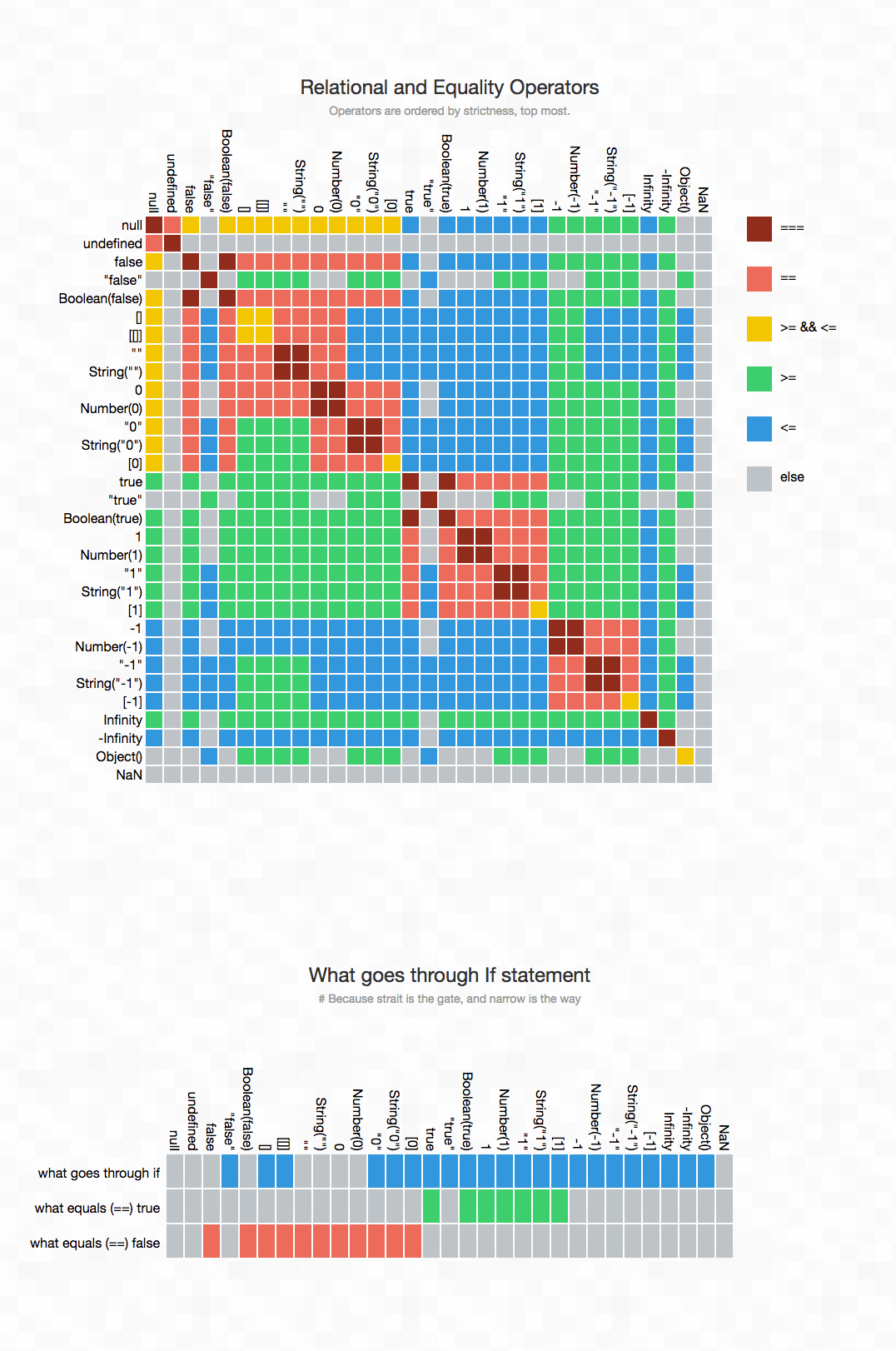if ( obj.a = = null ) {
// 上面这个判断相当于 obj.a = = = null || obj.a = = = undefined
// 上面这种简写方式 jQuery 中推荐的写法
}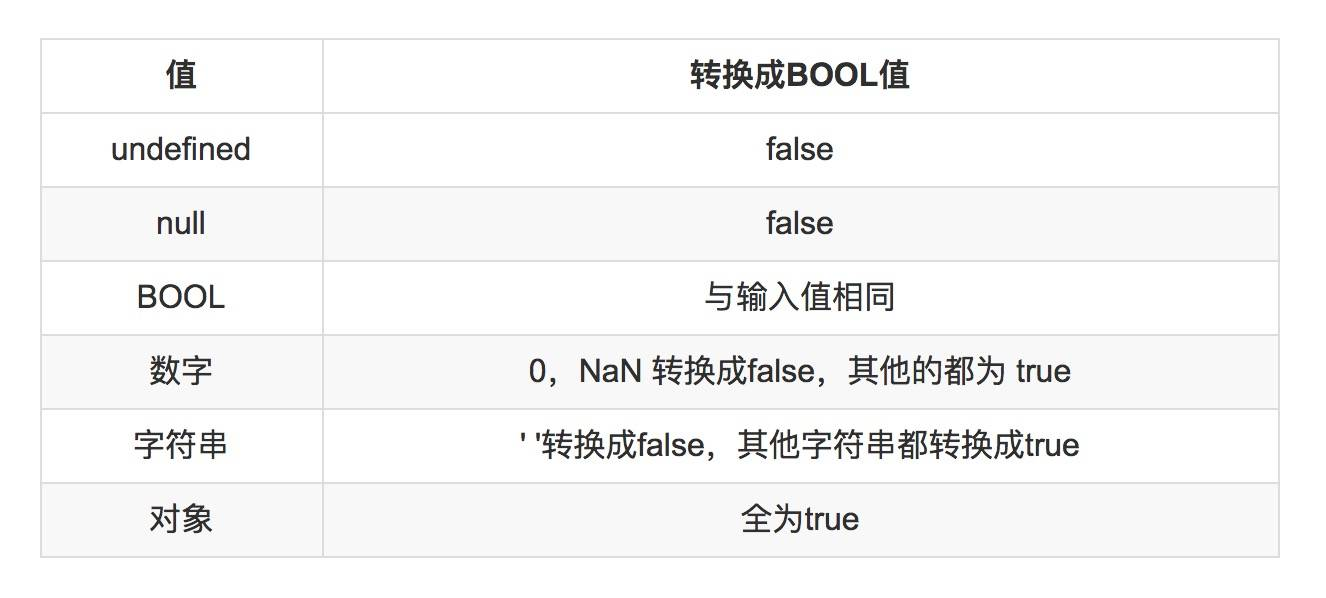### 三. Number

JavaScript 中所有的数字都只有一种类型，都被当做浮点数，JavaScript 内部会做优化，来区分浮点数组和整数。JavaScript 的数字是双精度的（64位），基于 IEEE 754 标准。``````
0.1 + 0.2 ;  // 0.300000000000004

( 0.1 + 0.2 ) + 0.3;    // 0.6000000000001
0.1 + ( 0.2 + 0.3 );    // 0.6

(0.8+0.7+0.6+0.5) / 4   // 0.65
(0.6+0.7+0.8+0.5) / 4   // 0.6499999999999999

``````

``````
( 8 + 7 + 6 + 5) / 4 / 10 ;  // 0.65
( 6 + 8 + 5 + 7) / 4 / 10 ;  // 0.65

``````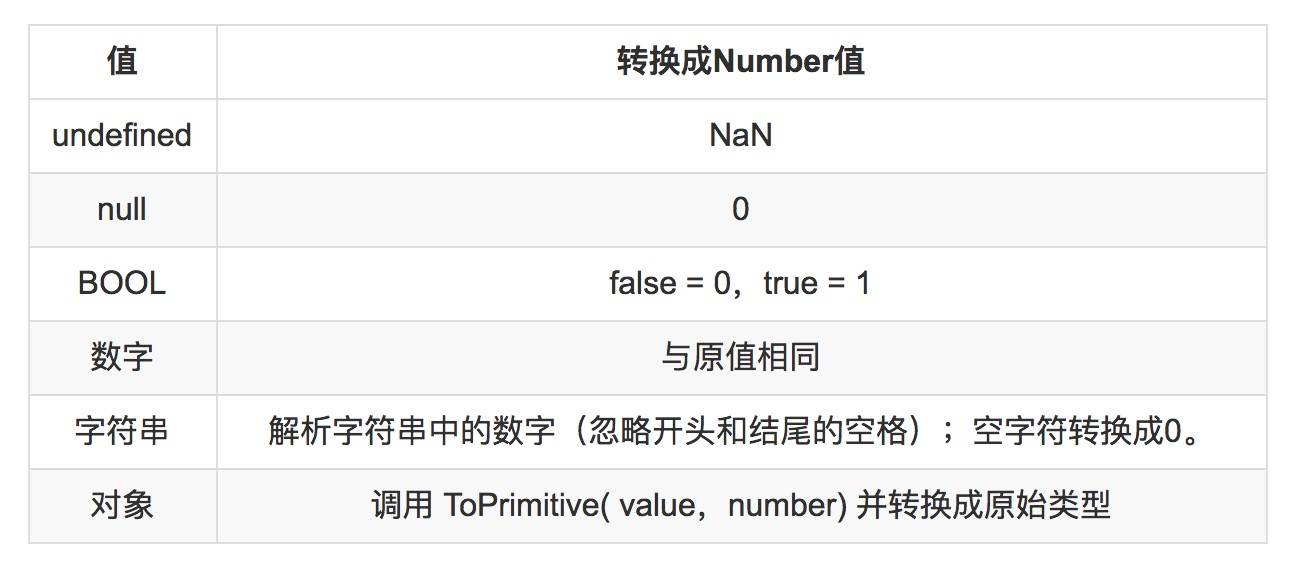1. 2个错误值：NaN 和 Infinity
2. 2个0，一个+0，一个-0。0是会带正号和负号。因为正负号和数值是分开存储的。
``````
typeof NaN
<"number"

``````

（吐槽：NaN 是 “ not a number ”的缩写，但是它却是一个数字）

NaN 是 JS 中唯一一个不能自身严格相等的值：

``````
NaN === NaN
<false

``````

``````
[ NaN ].indexOf( NaN )
<-1

``````

``````
function realIsNaN( value ){
return typeof value === 'number' && isNaN(value);
}

``````

``````
isNaN( 'halfrost' )
<true

``````

``````
function realIsNaN( value ){
return value !== value ;
}

``````

JS 中整型是有一个安全区间，在（ -2^53 ， 2^53）之间。所以如果数字超过了64位无符号的整型数字，就只能用字符串进行存储了。

``````
parseInt(1000000000000000000000000000.99999999999999999,10)
<1

``````

parseInt( str , redix? ) 会先把第一个参数转换成字符串:

``````
String(1000000000000000000000000000.99999999999999999)
<"1e+27"

``````

parseInt 不认为 e 是整数，所以在 e 之后的就停止解析了，所以最终输出1。

JS 中的 % 求余操作符并不是我们平时认为的取模。

``````
-9%7
<-2

``````

``````
function isOdd( value ){
return value % 2 === 1;
}

console.log(-3);  // false
console.log(-2);  // false

``````

``````
function isOdd( value ){
return Math.abs( value % 2 ) === 1;
}

console.log(-3);  // true
console.log(-2);  // false

``````

### 四. String

``````
'ä' < 'b'
<false

'á' < 'b'
<false

``````

### 五. Array

``````
new Array(2)  // 这里的一个数字代表的是数组的长度
<[ , , ]

new Array(2,3,4)
<[2,3,4]

``````

``````
var array = [1,2,3,4]
array.length
<4
delete array

array
<[1, ,3,4]
array.length
<4

``````

``````
var array = [1,2,3,4,56,7,8,9]
array.splice(1,3)
array
<[1, 56, 7, 8, 9]
array.length
<5

``````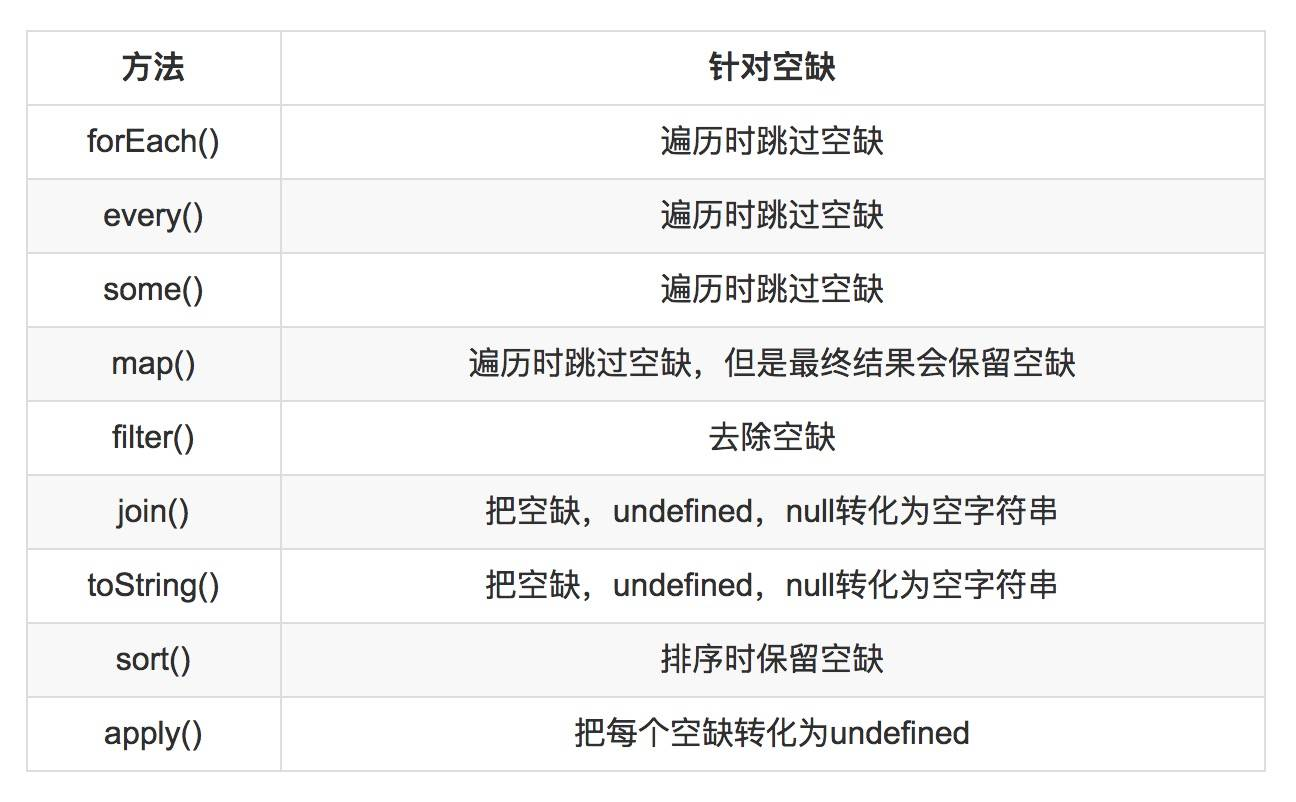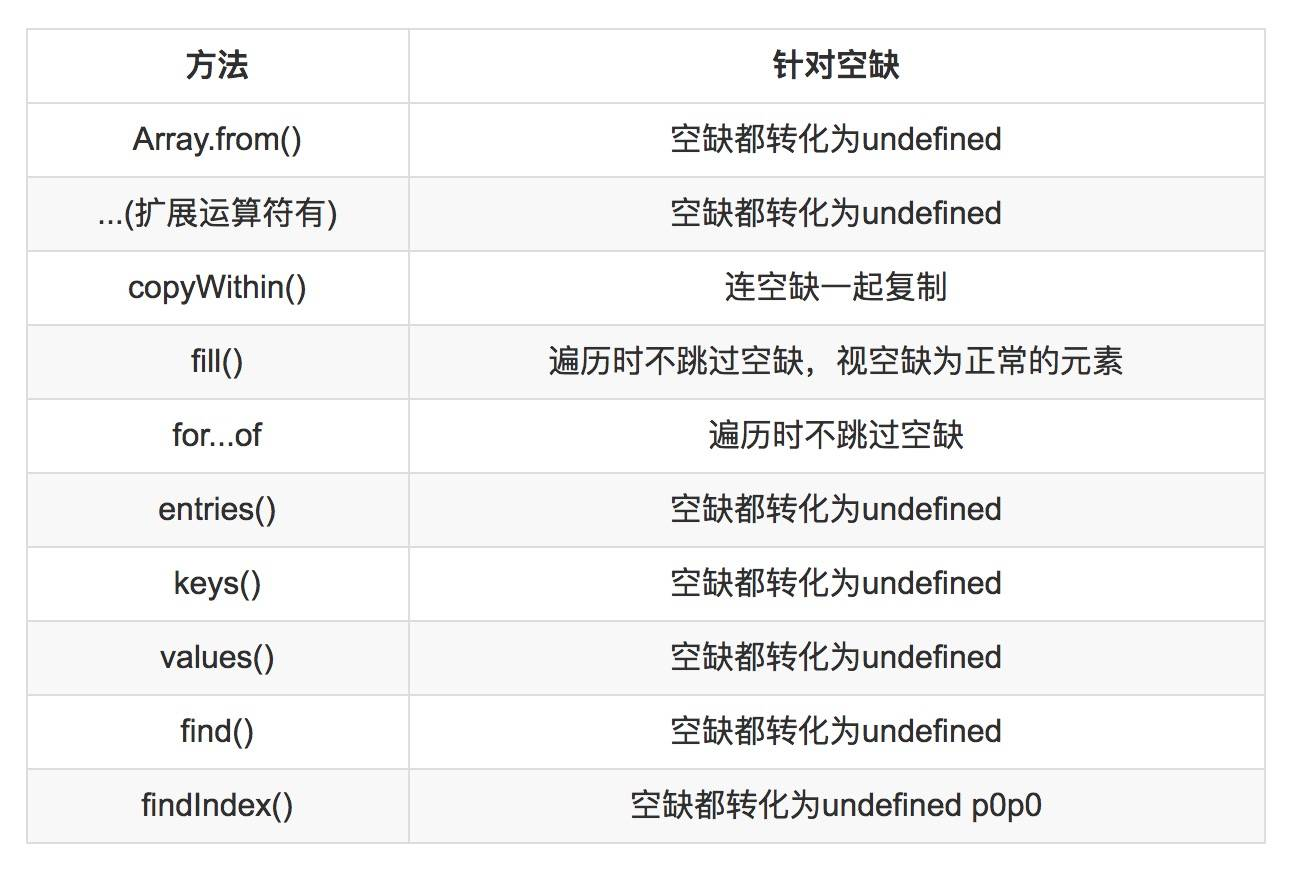### 六. Set 、Map、WeakSet、WeakMap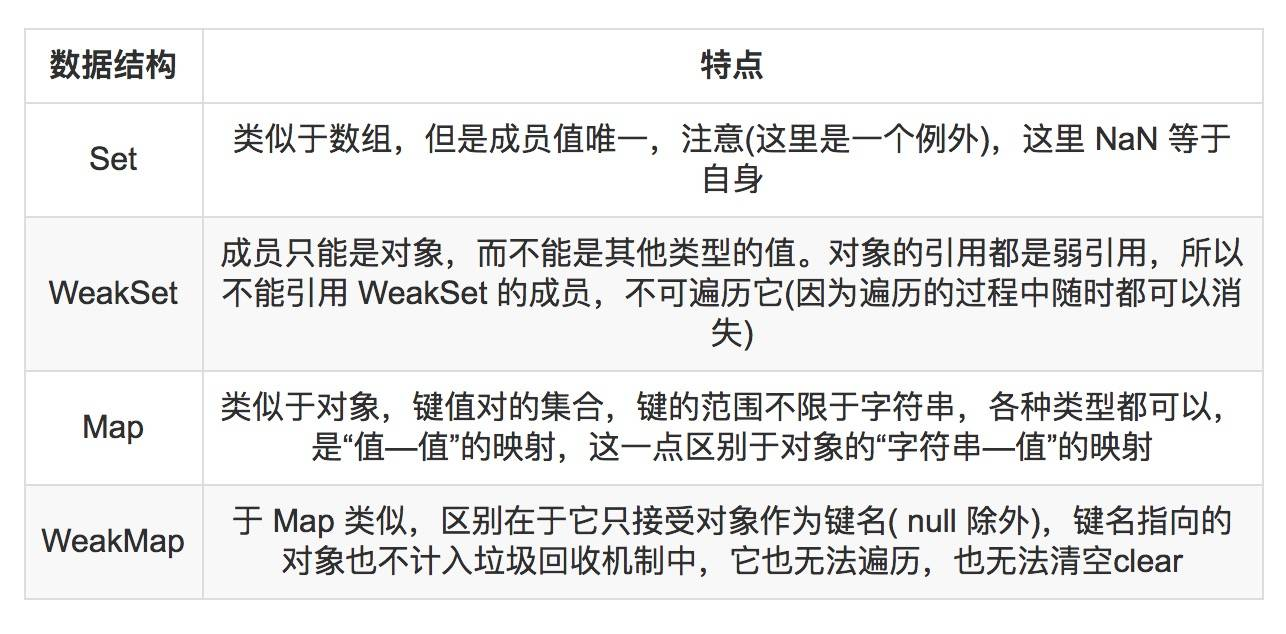### 七. 循环

``````
var scores = [ 11,22,33,44,55,66,77 ];
var total = 0;
for (var score in scores) {
total += score;
}

var mean = total / scores.length;

mean;

``````

``````
var scores = [ 1242351,252352,32143,452354,51455,66125,74217 ];

``````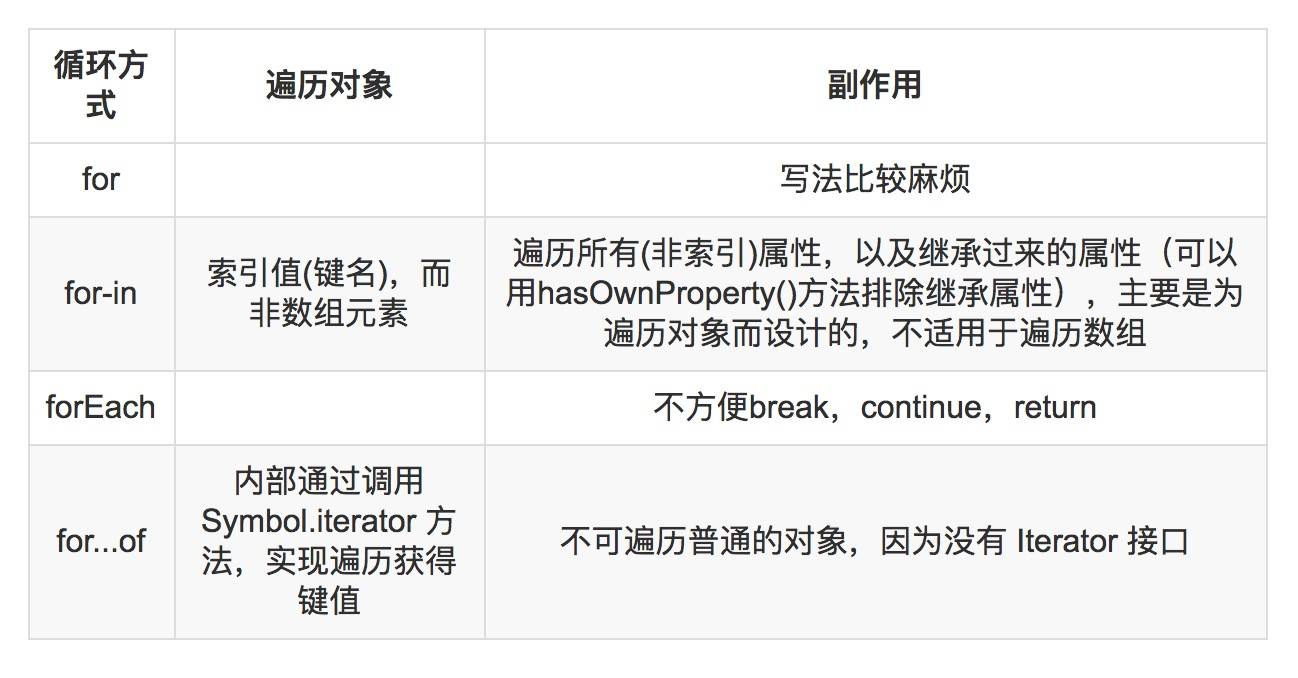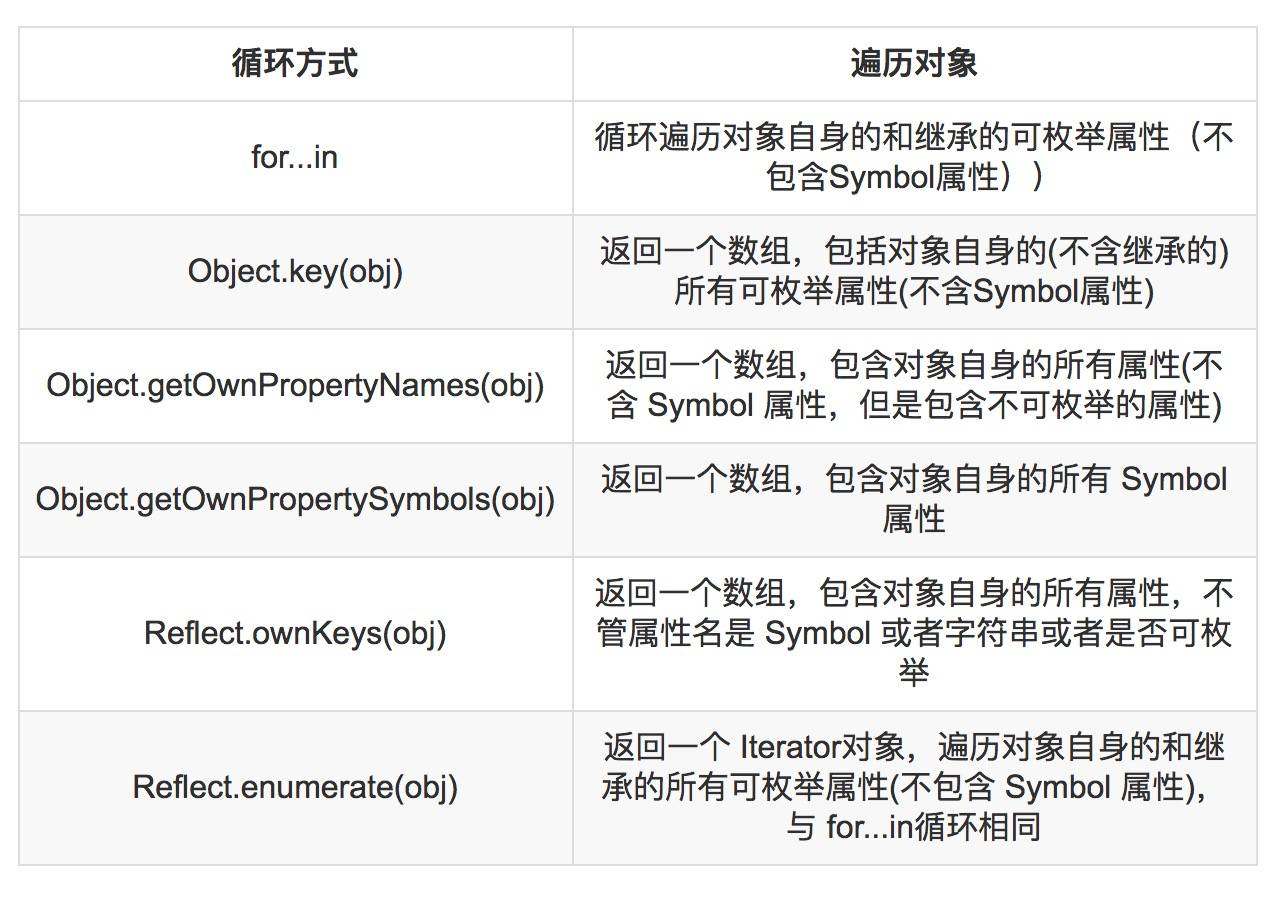### 八. 隐式转换 / 强制转换 带来的bug

``````
var formData = { width : '100'};

var w = formData.width;
var outer = w + 20;

console.log( outer === 120 ); // false;
console.log( outer === '10020'); // true

``````

### 十. 函数声明和变量声明的提升

``````
function foo() {
bar();
function bar() {
……
}
}

``````

var 变量也具有提升的特性。但是把函数赋值给变量以后，提升的效果就会消失。

``````
function foo() {
bar(); // error！
var bar = function () {
……
}
}

``````

JavaScript 支持词法作用域( lexical scoping )，即除了极少的例外，对变量 foo 的引用会被绑定到声明 foo 变量最近的作用域中。ES5中 不支持块级作用域，即变量定义的作用域并不是离其最近的封闭语句或代码块，而包含它们的函数。所有的变量声明都会被提升，声明会被移动到函数的开始处，而赋值则仍然会在原来的位置进行。

``````
function foo() {
var x = -10;
if ( x < 0) {
var tmp = -x;
……
}
console.log(tmp);  // 10
}

``````

``````
foo = 2;
var foo;
console.log( foo );

``````

``````
var foo;
foo = 2;
console.log( foo );

``````

``````
console.log( a );
var a = 2;

``````

``````
var foo;
console.log( foo );
foo = 2;

``````

``````
foo(); // 1
var foo;
function foo() {
console.log( 1 );
}
foo = function() {
console.log( 2 );
};

``````

``````
function foo() {
console.log( 1 );
}
foo(); // 1
foo = function() {
console.log( 2 );
};

``````

ES6 的这种做法也给 JS 带来了块级作用域，（在 ES5 中只有全局作用于和函数作用域），于是立即执行匿名函数（IIFE）就不在必要了。

### 十一. arguments 不是数组

arguments 不是数组，它只是类似于数组。它有length属性，可以通过方括号去访问它的元素。不能移除它的元素，也不能对它调用数组的方法。

``````
function callMethod(obj,method) {
var shift = [].shift;
shift.call(arguments);
shift.call(arguments);
return obj[method].apply(obj,arguments);
}

var obj = {
add:function(x,y) { return x + y ;}
};

``````

``````

Uncaught TypeError: Cannot read property 'apply' of undefined
at callMethod (<anonymous>:5:21)
at <anonymous>:12:1

``````

``````
function values() {
var i = 0 , n = arguments.length;
return {
hasNext: function() {
return i < n;
},
next: function() {
if (i >= n) {
throw new Error("end of iteration");
}
return arguments[i++];
}
}
}

var it = values(1,24,53,253,26,326,);
it.next();   // undefined
it.next();   // undefined
it.next();   // undefined

``````

``````
function values() {
var i = 0 , n = arguments.length,a = arguments;
return {
hasNext: function() {
return i < n;
},
next: function() {
if (i >= n) {
throw new Error("end of iteration");
}
return a[i++];
}
}
}

var it = values(1,24,53,253,26,326,);
it.next();   // 1
it.next();   // 24
it.next();   // 53

``````

### 十三. 函数中 this 的问题

``````
var halfrost = {
name:'halfrost',
friends: [ 'haha' , 'hehe' ],
sayHiToFriends: function() {
'use strict';
this.friends.forEach(function (friend) {
// 'this' is undefined here
console.log(this.name + 'say hi to' + friend);
});
}
}

halfrost.sayHiToFriends()

``````

``````
sayHiToFriends: function() {
'use strict';
var that = this;
this.friends.forEach(function (friend) {
console.log(that.name + 'say hi to' + friend);
});
}

``````

``````
sayHiToFriends: function() {
'use strict';
this.friends.forEach(function (friend) {
console.log(this.name + 'say hi to' + friend);
}.bind(this));
}

``````

``````
sayHiToFriends: function() {
'use strict';
this.friends.forEach(function (friend) {
console.log(this.name + 'say hi to' + friend);
}, this);
}

``````

1. 不能当做构造函数，不能使用 new 命令，因为没有 this，否则会抛出一个错误。
2. 不可以使用 argument 对象，该对象在函数体内不存在，非要使用就只能用 rest 参数代替。也不能使用 super，new.target 。
3. 不可以使用 yield 命令，不能作为 Generator 函数。
4. 不可以使用call()，apply()，bind()这些方法改变 this 的指向。

### 十四. 异步

1. 回调函数callback
2. 事件监听
3. 发布 / 订阅
4. Promise对象
5. Async / Await

(这个日记可能一直未完待续......)Previous Post

Next Post